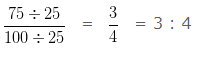# Convert Percentage to Ratio

### How to Convert Percentage to Ratio ?

Step I – In order Convert Percentage to Ratio, we need to divide the given percentage by 100.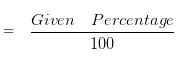Step II  – Reduce the Fraction obtained in Step I into its Simplest form.

Step III – Convert the Fraction ( obtained in step II ) into Ratio by replacing the slash mark with a colon.

### Convert Percentage to Ratio Examples

Example 1

Express 40 % as a ratio.

Explanation

In order Convert Percentage to Ratio, we need to divide the given percentage by 100.= 40/100

On Simplifying, 40/100

HCF of 40 and 100 is 20

Divide both 40 and 100 by their HCF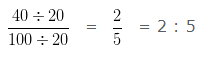Example 2

Express 20 % as a ratio.

Explanation

In order Convert Percentage to Ratio, we need to divide the given percentage by 100.= 20/100

On Simplifying, 20/100

HCF of 20 and 100 is 20

Divide both 20 and 100 by their HCF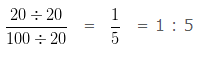Example 3

Express 35 % as a ratio.

Explanation

In order Convert Percentage to Ratio, we need to divide the given percentage by 100.= 35/100

On Simplifying, 35/100

HCF of 35 and 100 is 5

Divide both 35 and 100 by their HCF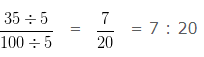Example 4

Express 75 % as a ratio.

Explanation

In order Convert Percentage to Ratio, we need to divide the given percentage by 100.= 75/100

On Simplifying, 75/100

HCF of 75 and 100 is 25

Divide both 75 and 100 by their HCF